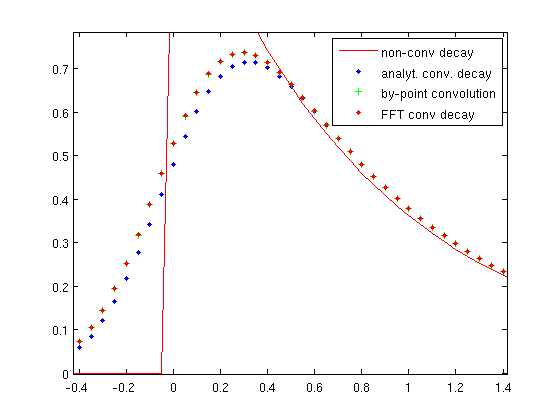# Analytical and numerical convolutions make pdf distinct

I attach a draft where two pdf-s are compared.
The first is an ordinary RooDecay convoluted with Gaussian (drawn in blue).
The second is the same exponential decay but introduced as a c-function. Then it is convoluted numerically with the same gaussian as the first decay (drawn in violet).
It’s obvious that the second PDF is shifted to the left. As far as I understand, the overall result should be independent of the way how convoluted PDF is produced. Correct me if i’m not right.
This distinction can be really misleading if one begins to mix pdf-s cnvoluted numerically and analytically.
My Root version is 5.30/02

P.S. I cant post this as a bug because my current ip is blacklistedinterp_prot.c (2.48 KB)

Dear developers!

Though I’m not able to close this bug by myself, i propose (an obvious!) solution.
Please check the shift of the final inverse FFT.
When I use RooNumConvPdf instead of FFT convolution, the result (=shift) is o.k.
I’ve simulated 3 ways of convolution with the help of Matlab (see pastebin.com/1kCfB5d0)
There is a subtle moment with this IFFT shift, but it can be adjusted and checked by observing the difference between analytical (or at least by-point) convolution and FFT convolution.

P.S. Soon I’m going to post here about another bug (may be feature?) concerning FFT convolution.Hi Dmitry,

It looks like you run into a finite resolution effect of the FFT convolution here - which calculated in a discrete sampling of the pdf. If the average in each bin does not perfectly line up with the bin center some migrations like you see can occur. The simplest thing is to use many more samplings, e.g. as follows

t.setBins(10000,“cache”) ;

to increase the numbers for FFT sampling from 100 to 10000. (This was also shown in the tutorial macro
root.cern.ch/root/html/tutorials … ion.C.html )

With this increasing sampling resolution FFT and numerical convolution agree very well (curves are right on top of each other). Note that this increase in sampling frequence does not increase the CPU time by much.

Wouter